Courses

# Oxidation And Reduction - Mole Concept Chemistry Notes | EduRev

## Physical Chemistry

Created by: Asf Institute

## Chemistry : Oxidation And Reduction - Mole Concept Chemistry Notes | EduRev

The document Oxidation And Reduction - Mole Concept Chemistry Notes | EduRev is a part of the Chemistry Course Physical Chemistry.
All you need of Chemistry at this link: Chemistry

Characteristics of Oxidation-Reduction
1. Oxidation or de-electronation is a process which liberates electrons.
2. Reduction or electronation is a process which gains electrons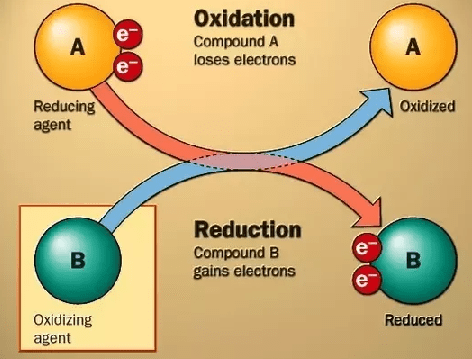electron movement in oxidation and reduction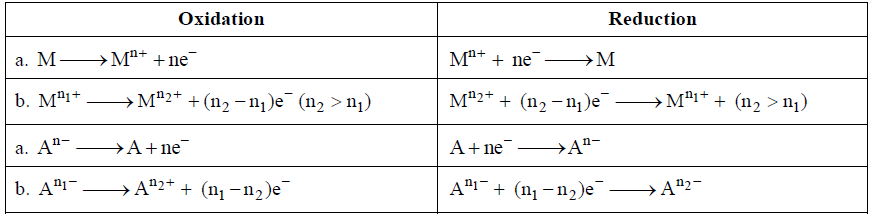3. Oxidant are substances which

• Oxidise other
• Are reduced themselves
• Show gain of electrons
• Show a decrease in oxidation number during a change.
• Have higher oxidation number in a conjugate pair of redox.

4. Reductants are the substances which

• Reduce other.
• Are oxidised themselves
• Show loss of electrons
• Show an increase in oxidation number during a change
• Have lower oxidation number in a conjugate pair of redox.

5. A redox change involves the process in which a reductant is oxidized to liberate electron, which are then taken up by an oxidant to get itself reduced.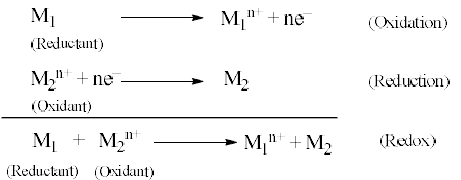6. A redox change occur simulataneously.

Types of Redox Reactions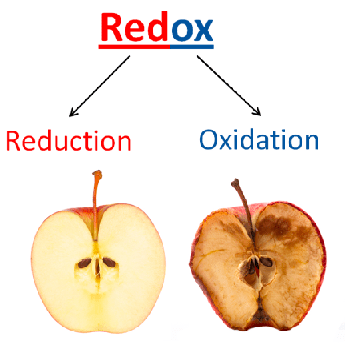redox meaning1. Inter-molecular redox reactions
Two substance reacts; one of them is oxidant and other is reductant, e.g.,

10FeSO4 + 2KMnO4 + 8H2SO4 → 2MnSO4 + 5Fe2 (SO)2 + K2SO4 + 8H2O
(reductant)         (Oxidant)

Or

2Fe2+ → (Fe3+)2 + 2e

And Mn7+ + 5e → Mn2+

2. Auto-redox or Disproportionation
The same element of a substance is oxidized and reduced as well, e.g.,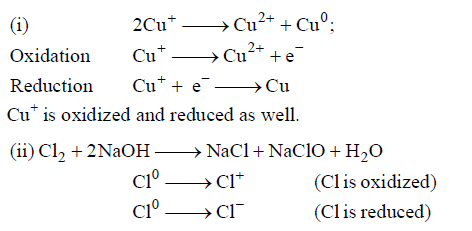3. Intra-molecular redox reactions
One element of a compound is oxidized and the other element of the same compound is reduced, e.g.,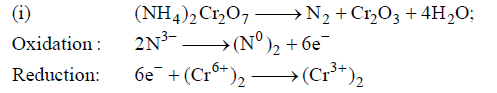Oxidation Number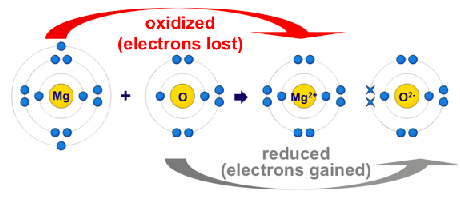electron movement decides the oxidation numberOxidation number of an element in a particular compound represents the number of electrons lost or gained by an element during is change from free state into that compound. Oxidation number is given positive sign if electrons are lost. Oxidation number is given negative sing if electrons are gained. Oxidation number represents real charge in case of ionic compounds, however, in compounds it represents for imaginary charge.

The rules of deriving oxidation number
Following arbitrary rules have been adopted to derive oxidation number of elements on the basis of periodic properties of elements.
(i) In uncombined state or free state, oxidation number of an element is zero
(ii) In uncombined state oxidation number of

• F is always –1
• O is –2; in peroxides (—O—O—), it is –1. However, in F2O, it is +2. In superoxide, it is -1/2
• H is +1; In ionic hydrides it is –1
• Metals is positive except in some metal hydrides as CuH(Cu1–) and [Rh(CO)4], both metals have –ve oxidation number.
• Alkali metals (IA, e.g., Li, Na, K, Rb, Cs, Fr) is always +1
• Alkaline earth metals (IIA, e.g., Be, Mg, Ca, Sr, Ba, Ra) is always +2
• Halogens in halides is always –1
• Sulphur in sulphides is always –2]

(iii) The algebraic sum of all the oxidation numbers of elements in a compound is equal to zero, e.g., K2MnO4.
2 × (oxidation number of K) + (oxidation number of Mn) + 4 × (oxidation number of O) = 0
(iv) The algebraic sum of all the oxidation numbers of elements in a radical is equal to net charge on that radical, e.g., C2O42- .
2 × (oxidation number of C) + 4 × (oxidation number of O) = –2
(v) Oxidation number of can be zero, +ve, –ve integer or fraction.
(vi) Maximum oxidation number of an element (except O and F) = group number.
(vii) Minimum oxidation number of an element (except metals) = group number –8
(viii) Variable oxidation number is most commonly shown by transition elements as well as by p-block elements.

Iodimetric titration
Iodine is a moderately strong oxidising agent and can be used to titrate reducing agent but their number is limited.
The redox titrations involving iodine solution as an oxidant, e.g., Na2S2O3 vs. I2 are called iodometric titrations. However I2 being sparingly soluble in water and therefore solution of Iused for iodometric titrations is prepared in presence of KI. Presence of KI increases the solubility of I2 in water due to complex formation.
The reaction is:

2Na2S2O3 + I2  → Na2S4O6 + 2NaI

Redox changes are: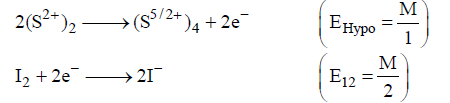Indicator: I2 acts as self-indicator with starch solution. The disappearance of blue colour of starch –I2 indicates the end point of titration.

Iodometric titrations
Iodide ion is a weak reducing agent and will reduce strong oxidising agents. The redox change involving liberated iodine as an oxidant e.g., CuSOvs. Hypo in presence of KI are called iodometric titration.
The reaction are: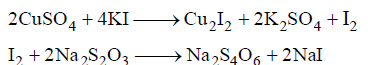Redox changes are like iodometry. However the iodometric titrations should be performed rapidly to minimise oxidation of iodide by air.

Example: A 1.100 g sample of copper ore is dissolved and the Cu2+ of is treated with excess KI. The liberated I2 requires 12.12 mL of 0.10 M Na2SOsolution for titration. What is % copper by mass in the ore: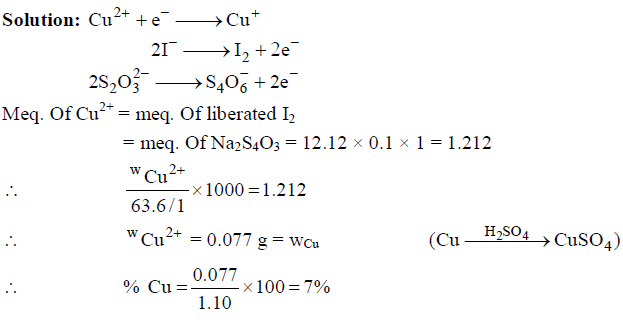Offer running on EduRev: Apply code STAYHOME200 to get INR 200 off on our premium plan EduRev Infinity!

,

,

,

,

,

,

,

,

,

,

,

,

,

,

,

,

,

,

,

,

,

;